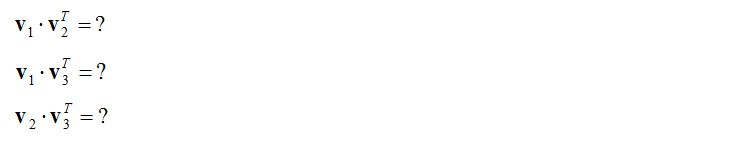Engineering Math - Quick Reference                                 Home : www.sharetechnote.com Matrix Orthogonalization - Gram Schmidt Process   Matrix Orthogonalization is a process of deriving a Orthogonal Matrix from a non-orthogonal matrix. This can be applied to both column vectors and row vectors as illustrated below. (Note that the derived matrix may maintain a certain properties of the orignal matrix, but they are not exactly same).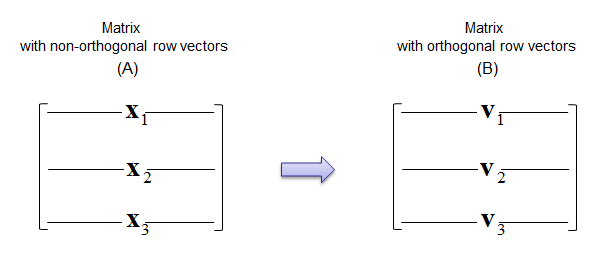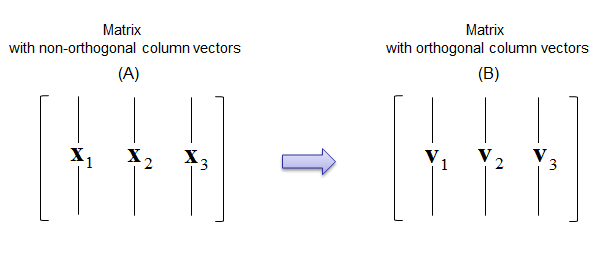Following is the process showing you derives the orthogonal matrix. If you just want to have algorithm, just copy this one as you need. But if you really want to understand the meaning of each step and how this process works, refer to Vector projection onto a Line first. Then these procedure would make more sense to you.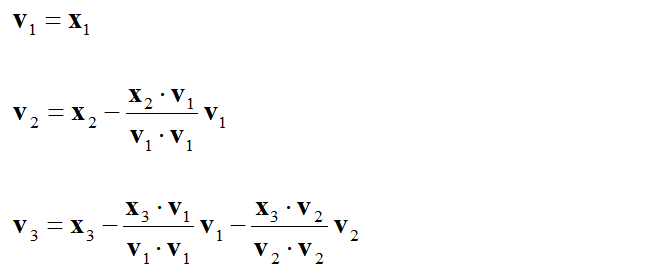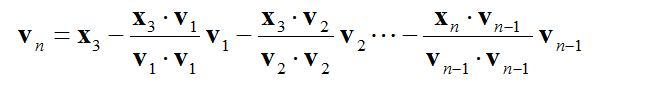If you look into the mathematical meaning of each step, it can be described as shown below.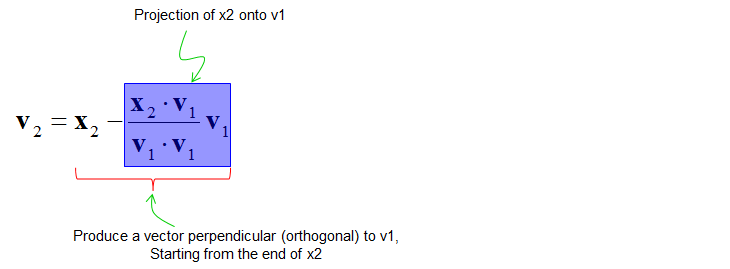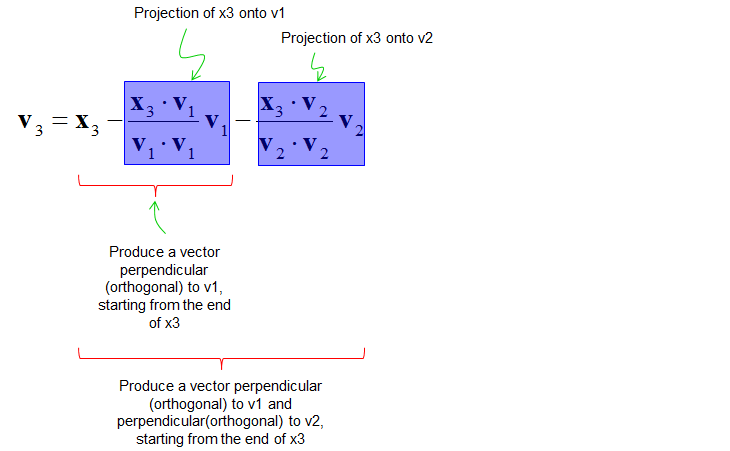If you look into the concept of vector projection onto a line, you would easily understand that this process can be described as shown below.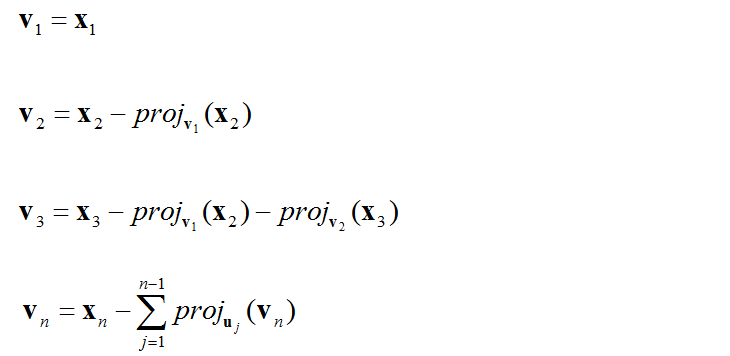Example >   Let's assume that we are given a matrix as follows and assume that we want to orthogonalize the row vectorsAt first, let's take out each row as vectors as follows.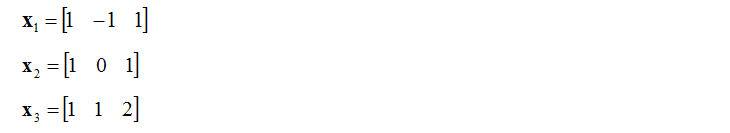Now just plug in these vectors into each procedures and you will get the following result. (Note that the second vector in each inner product is transposed. This is to make inner product possible and produce a scalar value)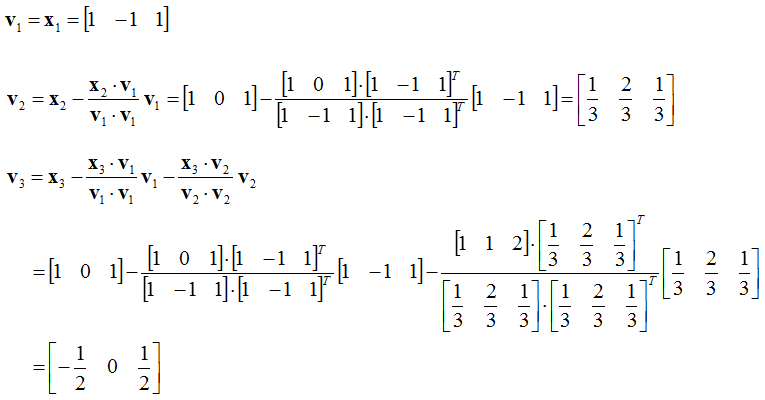Now we have completed the calculation. Then you may ask how we can be sure that the answer is correct ? One way to prove it is to draw the resulting vectors into a coordinate and check if the angles between each vector are 90 degree.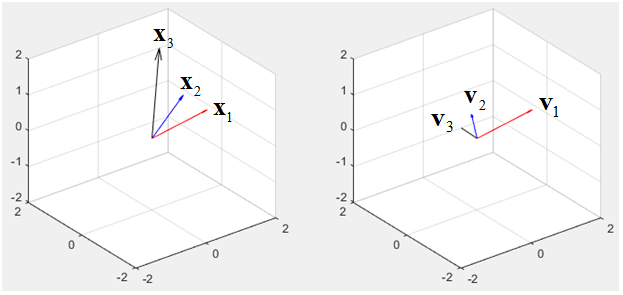However this kind of graphical method can be an easy and quick method in 2 vectors (2 Dimensional), but in 3 vectors, it is difficult to measure the angles between vectors correctly. If it goes more than 3, it is impossible to apply this kind of graphical method.   More accurate way is to take the innerproduct of all the two combination from the resulting matrix. In case of this example, take the following inner product. If you get '0' for all of these, it means that you derived a correct orthogonal matrix.